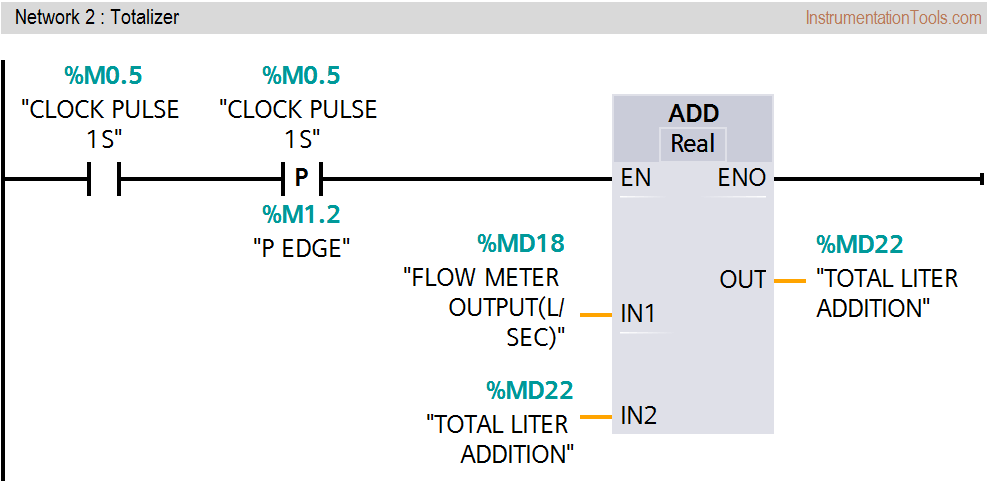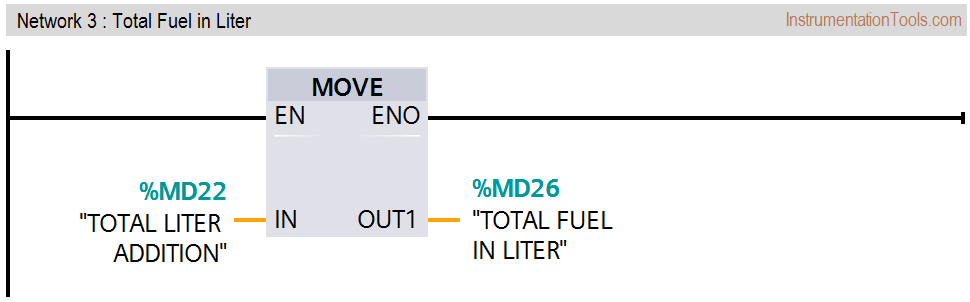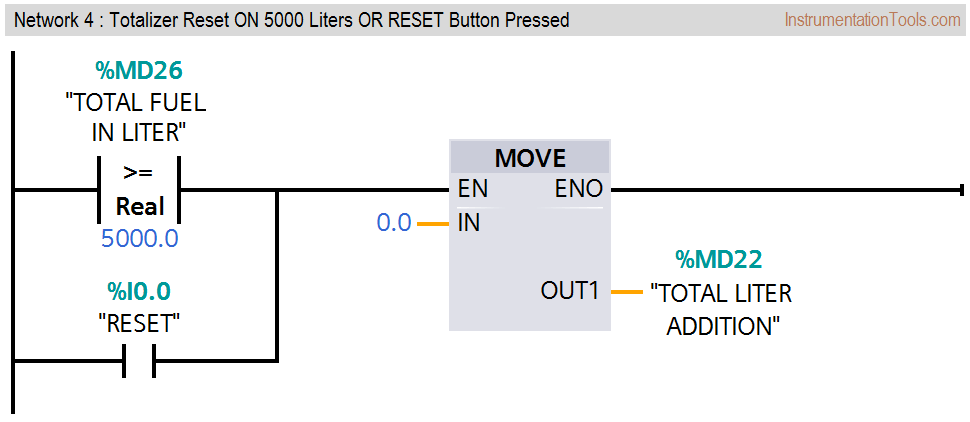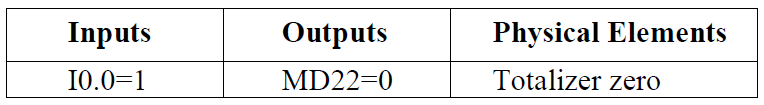Home » PLC Program for Flow Totalizer
PLC Tutorials

# PLC Program for Flow Totalizer

Make PLC program to implement totalizer for flow meter. The flow meter has 4-20mA output that represents 0 to 100 liters/hour fuel flow in a pipe.

By using this logic, we can calculate total fuel passed from the pipe. When totalizer value will reach to 5000 liters, then automatically it should be reset or we can reset the value using RESET button.

## PLC Program for Flow Totalizer### Problem Solution

• We can solve this problem by simple logic. Here we consider a flow meter for measuring the fuel with maximum flow rate of 100 liters/hour.
• Here we will convert this flow rate from L/H to L/Sec by using DIV instruction for calculation.
• After then by using 1 second clock pulse, we will store this value in other memory location and every second new value will be added & updated.
• Here for example we consider max value for totalizer is 5000 liters so after this value totalizer should be RESET.
• So we will compare this value with actual value and reset it automatically or we will provide RESET button to reset the totalizer value.

### List of Inputs/Outputs

#### Inputs List

• Reset :- I0.0

#### M Memory

• M0.5 :- 1 second (1s) clock pulse
• M1.2 :- Positive edge of clock pulse
• MD10 :- Memory word for final output (L/H) of flow meter
• MD18 :- Memory word for final output (L/Sec) of flow meter
• MD26 :-Total fuel in liter

### PLC Ladder diagram for Totalizer### Program Description

In this problem we will consider S7-300 PLC and TIA portal software for programming.

#### Network 1 :-

Here we have taken final output value of the flow meter in L/H (MD10). By using DIV instruction we converted L/H flow into L/sec and final value stored in MD18.

#### Network 2 :-

Here clock pulse of 1s (M0.5) will add value every second and store the result in memory word MD22.

#### Network 3 :-

Here we moved value of MD22 in MD26 (total fuel in liter) for display purpose.

#### Network 4 :-

In this network we need to reset totalizer. If total fuel is greater than 5000 (5000 value is for example purpose, it is depended on flow meter configuration & it’s range ) then totalizer count should be zero automatically or we can reset by pressing RESET button (I0.0).

Note: – Above logic is for explanation purpose only. Here we have considered only final output of the scaling, so we have not mentioned 4-20mA scaling in the logic.

### Runtime Test Cases#### 1 commentSeptember 6, 2019 at 7:20 am

hi admin, I0.0 receives the signal from the flow meter. so how to get the signal of I0.0 to MD10. I don’t understand that place

This website uses cookies to improve your experience. We'll assume you're ok with this, but you can opt-out if you wish. Accept Read More

WordPress Image Lightbox

Send this to a friend